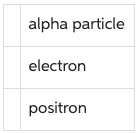# Problem: What particle is emitted in the radioactive decay 2714Si →  2713Al + ?What particle is emitted in the radioactive decay ?

###### FREE Expert Solution

In this problem, we'll write a balanced expression of the mass number and the number of protons, then determine the unknown.

Part A

27 = 27 + y

y = 0

93% (490 ratings)###### Problem Details

What particle is emitted in the radioactive decay 2714Si →  2713Al + ?

What particle is emitted in the radioactive decay ?